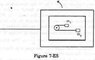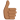# What causes the acceleration?

• Mastermind01
In summary, a table with smooth horizontal surface placed in a cabin moving in a circular path with a large radius R has two masses, m and 2m, connected by a string over a small pulley attached to the table. When the system is released from rest, the masses experience an initial acceleration 'a' with respect to an observer inside the cabin. The tension in the string, T, is the cause of the centripetal force that creates the acceleration. The concept of centrifugal force can also be used to explain the motion, but it is not recommended by certain sources. To calculate the initial acceleration without using centrifugal force, one would need to consider the moment of

## Homework Statement

A table with smooth horizontal surface is placed in a cabin which moves in a circle of a large radius R (figure attached). A smooth pulley of small radius is fastened to the table. Two masses m and 2m placed on the table are connected through a string going over the pulley Initially the masses are held by a person with the strings" along the outward radius and then the system is released from rest (with respect to the cabin). Find the magnitude of the initial acceleration of the masses as seen from the cabin and the tension in the string.

## Homework Equations

centripetal acceleration = $$\frac{v^2}{r } = \omega^2r$$

## The Attempt at a Solution

Assuming an acceleration of a of one of the masses attached to the pulley produces the two equations:

$$T = m(\omega^2R + a)$$

and

$$T = 2m(\omega^2R - a)$$

which solves the question and gives the requisite answer.
However my question is what causes the acceleration 'a' in the first place ? The only force acting on the masses is the tension 'T' which I think is the cause of the centripetal force.

Thanks.

#### Attachments

•image02072015384.jpg
3.5 KB · Views: 441
As you know F=ma. So applying a force causes a mass to feel an acceleration. So with regards to what causes the acceleration in your case, it is the centripetal force (Yes that is T) itself that causes the centripetal acceleration. Hope this helps.

DarkMatter5 said:
As you know F=ma. So applying a force causes a mass to feel an acceleration. So with regards to what causes the acceleration in your case, it is the centripetal force (Yes that is T) itself that causes the centripetal acceleration. Hope this helps.

To someone outside the cabin the tension T causes the centripetal acceleration but the 'a' that I got is the acceleration of the mass with respect to an observer inside the cabin . What I want to know is what causes the acceleration 'a' with respect to the cabin.

To clarify: 'a' isn't the centripetal acceleration , it is the acceleration with respect to the inside of the cabin.

Oh I see. But the force is still caused by the same tension inside the cabin. So the acceleration should be caused by the Tension. What am I missing here?

DarkMatter5 said:
Oh I see. But the force is still caused by the same tension inside the cabin. So the acceleration should be caused by the Tension. What am I missing here?

Which is what I thought - but I can't figure out why would the tension do that (create the 'a') ?

Tension is the inward force. Since the tension is a force then it would create an acceleration. As I said before, forces create acceleration. That is what forces do.

DarkMatter5 said:
Tension is the inward force. Since the tension is a force then it would create an acceleration. As I said before, forces create acceleration. That is what forces do.

My understanding of tension is probably flawed here - but I always thought tension to be the force exerted by a string on a mass in opposition to another force. In most problems (mass hanging from rope , a pulley system , friction on a table) there is an opposing force (weight of the body , static/kinetic friction) which creates the tension in the rope. However in the situation inside the cabin, no such force is acting on the body. From the ground there is a component of acceleration in the direction of the tension, however with respect to an observer inside the cabin there is no force acting on it, so why would the mass in the pulley system move?

Okay I understand what you are saying here.

You are mostly correct in your understanding of tension. Tension is a force exerted by the string on the mass towards the centre (in this case the centripetal force).
Also you are correct that no force is acting against the tension in this case.
But you seem to not understand how circular motion works in regard to what is acting against the tension. Tension is a normal, regular force like any other force (friction, you pushing on a wall, etc). Forces do not always have to work against another external force. For example when I push a wall, the wall pushes back but I don't need another person on the other side of the wall to push it. The wall can do that itself.
Hence, the tension in the rope is working against the mass pulling against the rope in the opposite direction.
Why does the mass pull the rope during circular motion? In the reference frame of inside the cabin there seems to be an outward force called "centrifugal" force. This is however ficticious. The velocity of the mass tangental to the circular path and the inertia of the mass itself creates this effect.

So in conclusion, the tension is pulling against the inertia and tangental velocity of the mass. This tension force creates the acceleration.

Any questions?

•Mastermind01
Thanks for the explanation. Centrifugal force certainly does solve the problem however I was hoping to do it without using centrifugal force as Sears/Zhemansky/Freedman/Young strongly discourages using it to analyse systems.

Yes because it is a ficticious force. Use the components of the tangental velocity and inertia instead. That is real.
No problem. Happy to help.

DarkMatter5 said:
Yes because it is a ficticious force. Use the components of the tangental velocity and inertia instead. That is real.
No problem. Happy to help.

Sorry. I don't think I get you there. How would I go about doing that (without using centrifugal force)?

I don't think the question asked you to do that. From what I see it is asking for the centripetal acceleration.
What you asked is much more complicated.
You would have to, I think, take the moment of inertia (I), angular acceleration (α) and torque(τ) of the system into consideration.
I=mr2
τ=Iα

•Mastermind01
DarkMatter5 said:
I don't think the question asked you to do that. From what I see it is asking for the centripetal acceleration.
What you asked is much more complicated.
You would have to, I think, take the moment of inertia (I), angular acceleration (α) and torque(τ) of the system into consideration.
I=mr2
τ=Iα

I think there's no way to do this other than centrifugal force at this level. Torque and rotational mechanics come later in the book so irrelevant to the question. Thanks for all your help though..

No problem.